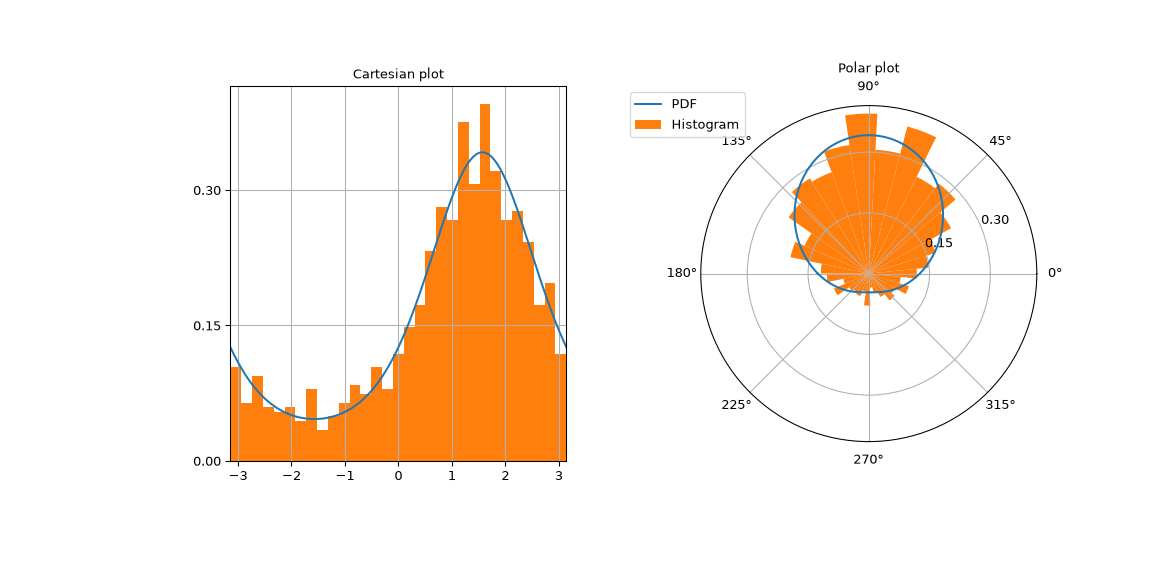# scipy.stats.vonmises_line¶

scipy.stats.vonmises_line = <scipy.stats._continuous_distns.vonmises_gen object>[source]

A Von Mises continuous random variable.

As an instance of the rv_continuous class, vonmises_line object inherits from it a collection of generic methods (see below for the full list), and completes them with details specific for this particular distribution.

Notes

The probability density function for vonmises and vonmises_line is:

$f(x, \kappa) = \frac{ \exp(\kappa \cos(x)) }{ 2 \pi I_0(\kappa) }$

for $$-\pi \le x \le \pi$$, $$\kappa > 0$$. $$I_0$$ is the modified Bessel function of order zero (scipy.special.i0).

vonmises is a circular distribution which does not restrict the distribution to a fixed interval. Currently, there is no circular distribution framework in scipy. The cdf is implemented such that cdf(x + 2*np.pi) == cdf(x) + 1.

vonmises_line is the same distribution, defined on $$[-\pi, \pi]$$ on the real line. This is a regular (i.e. non-circular) distribution.

vonmises and vonmises_line take kappa as a shape parameter.

The probability density above is defined in the “standardized” form. To shift and/or scale the distribution use the loc and scale parameters. Specifically, vonmises_line.pdf(x, kappa, loc, scale) is identically equivalent to vonmises_line.pdf(y, kappa) / scale with y = (x - loc) / scale. Note that shifting the location of a distribution does not make it a “noncentral” distribution; noncentral generalizations of some distributions are available in separate classes.

Examples

>>> from scipy.stats import vonmises_line
>>> import matplotlib.pyplot as plt
>>> fig, ax = plt.subplots(1, 1)


Calculate the first four moments:

>>> kappa = 3.99
>>> mean, var, skew, kurt = vonmises_line.stats(kappa, moments='mvsk')


Display the probability density function (pdf):

>>> x = np.linspace(vonmises_line.ppf(0.01, kappa),
...                 vonmises_line.ppf(0.99, kappa), 100)
>>> ax.plot(x, vonmises_line.pdf(x, kappa),
...        'r-', lw=5, alpha=0.6, label='vonmises_line pdf')


Alternatively, the distribution object can be called (as a function) to fix the shape, location and scale parameters. This returns a “frozen” RV object holding the given parameters fixed.

Freeze the distribution and display the frozen pdf:

>>> rv = vonmises_line(kappa)
>>> ax.plot(x, rv.pdf(x), 'k-', lw=2, label='frozen pdf')


Check accuracy of cdf and ppf:

>>> vals = vonmises_line.ppf([0.001, 0.5, 0.999], kappa)
>>> np.allclose([0.001, 0.5, 0.999], vonmises_line.cdf(vals, kappa))
True


Generate random numbers:

>>> r = vonmises_line.rvs(kappa, size=1000)


And compare the histogram:

>>> ax.hist(r, density=True, histtype='stepfilled', alpha=0.2)
>>> ax.legend(loc='best', frameon=False)
>>> plt.show()Methods

 rvs(kappa, loc=0, scale=1, size=1, random_state=None) Random variates. pdf(x, kappa, loc=0, scale=1) Probability density function. logpdf(x, kappa, loc=0, scale=1) Log of the probability density function. cdf(x, kappa, loc=0, scale=1) Cumulative distribution function. logcdf(x, kappa, loc=0, scale=1) Log of the cumulative distribution function. sf(x, kappa, loc=0, scale=1) Survival function (also defined as 1 - cdf, but sf is sometimes more accurate). logsf(x, kappa, loc=0, scale=1) Log of the survival function. ppf(q, kappa, loc=0, scale=1) Percent point function (inverse of cdf — percentiles). isf(q, kappa, loc=0, scale=1) Inverse survival function (inverse of sf). moment(n, kappa, loc=0, scale=1) Non-central moment of order n stats(kappa, loc=0, scale=1, moments=’mv’) Mean(‘m’), variance(‘v’), skew(‘s’), and/or kurtosis(‘k’). entropy(kappa, loc=0, scale=1) (Differential) entropy of the RV. fit(data) Parameter estimates for generic data. See scipy.stats.rv_continuous.fit for detailed documentation of the keyword arguments. expect(func, args=(kappa,), loc=0, scale=1, lb=None, ub=None, conditional=False, **kwds) Expected value of a function (of one argument) with respect to the distribution. median(kappa, loc=0, scale=1) Median of the distribution. mean(kappa, loc=0, scale=1) Mean of the distribution. var(kappa, loc=0, scale=1) Variance of the distribution. std(kappa, loc=0, scale=1) Standard deviation of the distribution. interval(alpha, kappa, loc=0, scale=1) Endpoints of the range that contains fraction alpha [0, 1] of the distribution